Related Articles

# Venn Diagram

• Last Updated : 26 May, 2021

Math is a subject where data plays a very important role and representing data in the easiest format is also very important. The ways to represent data in order to make it easy to understand are graphs, charts, diagrams. They not only make the Data look neat but also make it very easy to remember and understand properly, one of those ways is to represent the data through Venn Diagram.

### What is Venn Diagram?

Venn Diagrams are used to represent the groups of data into circles, if the circles are overlapping, some elements in the groups are common, if they are not overlapping, there is nothing common between the groups or sets of the data. The sets are represented as circles and the circles are shown inside the rectangle which is representing the Universal set. Universal set contains all the circles since it has all the elements present involving all the sets.

John Venn, a famous logician gave the concept of diagrams in 1918, this is why the diagrams are named Venn diagrams. There are several benefits of Venn Diagrams,

• Venn diagram is used for the classification of data belonging to the same category but different sub-categories.
• The comparison of the different data becomes easier through the Venn diagram, also the relationship between the data.
• Grouping the information and finding similarities and differences among them becomes easy.
• The different unknown parameters can be easily understood and found out with the help of Venn diagrams.

### Drawing Venn Diagram

In order to draw a Venn diagram, first, understand the type of symbols used in sets. Sets can be easily represented on the Venn diagram and the parameters are easily taken out from the diagram itself, formula is not even required.

Symbols:

Union of a Set⇢ ∪

Intersection of a Set⇢ ∩

Compliment of a Set A ⇢ A’ or Ac

The above symbols are used while drawing and showing the relationship among sets. In order to draw Venn diagram,

Step 1: Start by drawing a Rectangle showing the Universal Set.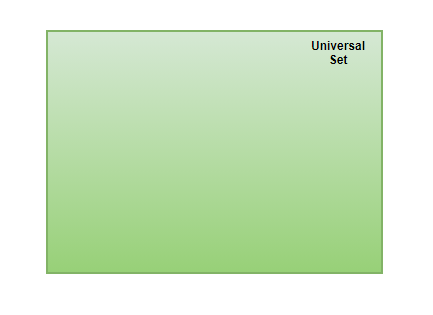Universal Set

Step 2: According to the number of sets given and the relationship between/among them, draw different circles representing different Sets.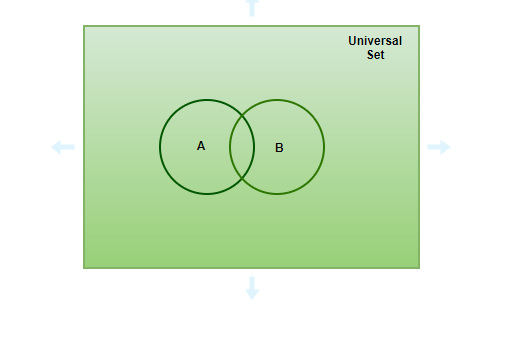Two-set Venn diagram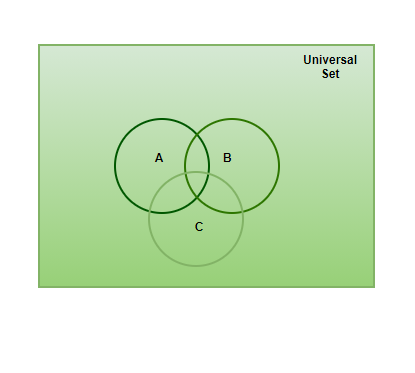Three-set Venn diagram

### Operation on Sets

There are different operations that can be done on sets in order to find the possible unknown parameter, for example, if two sets have something in common, their intersection is possible. Let’s look at the basic operations done on sets and how they look on the Venn diagram,

Complement of a set

Complementing a set means finding the value of the data present in the Universal set other than the data of the set.

n(A’) = U- n(A)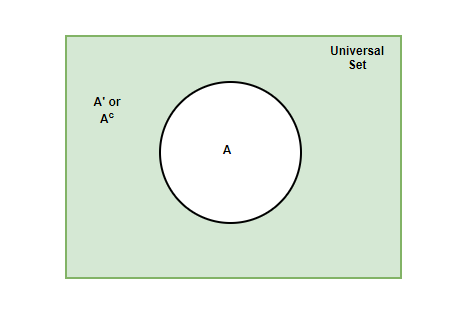Union of sets

Union of two or more than two sets represents the data of the sets without repeating the same data more than once, it is shown with the symbol ⇢∪.

n(A∪ B) = {a: a∈ A OR a∈ B}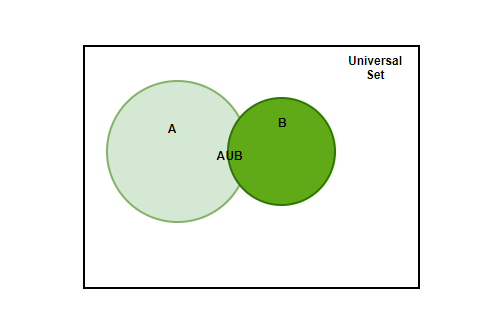Properties:

• A∪ B= B∪ A ⇢ [Commutative property]
• A∪ (B∪ C)= (A∪ B) ∪ C ⇢ [Associative property]
• A ∪ U= U
• A ∪ A’= U
• A ∪ A= A

Intersection of sets

Intersection of two or more than two sets means extracting only the amount of data that is common between/among the sets. The symbol used for the intersection⇢ ∩.

n(A∩ B)= {a: a∈ A and a∈ B}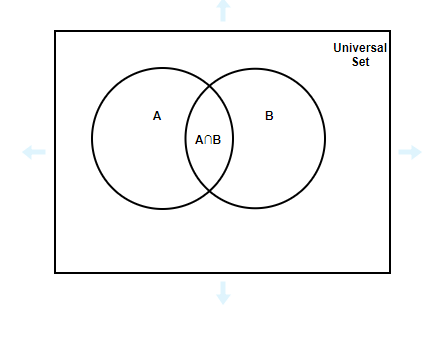Properties:

• A∩ B= B∩ A ⇢ [Commutative property]
• (A∩ B) ∩ C= A∩ (B ∩ C) ⇢ [Associative property]
• A∩A= A
• A ∩ U= A
• A∩ A’= φ

Representation of Three-set Venn diagram: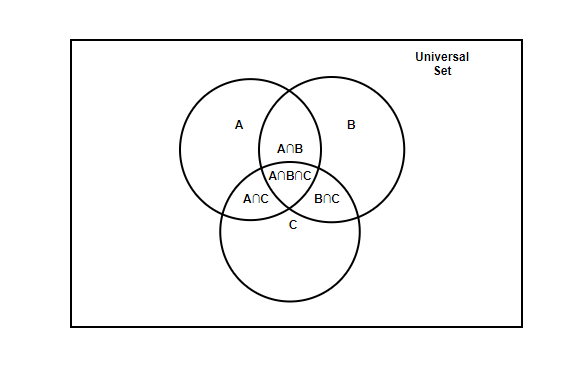### Sample Problems

Question 1: Set A= {1, 2, 3, 4, 5} and U= {1, 2, 3, 4, 5, 6, 7, 8, 9, 10}.

Represent A’ or Ac on Venn diagram.

Solution:

Venn Diagram for A’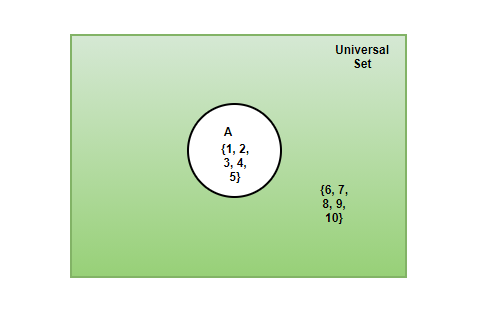Question 2: In a Group of people, 50 people either speak Hindi or English, 10 prefer speaking both Hindi and English, 20 prefer only English. How many people prefer speaking Hindi? Explain both by formula and by Venn diagram.

Solution:

According to formula,

n(H∪E) = n(H) + n(E) – n(H∩E)

Both English and Hindi speakers, n(H∩E) = 10

English speakers, n(E)= 20

Either Hindi or English, n(H∪E)= 50

50= 20+ n(H) – 10

n(H)= 50 – 10

n(H)= 40

From Venn Diagram,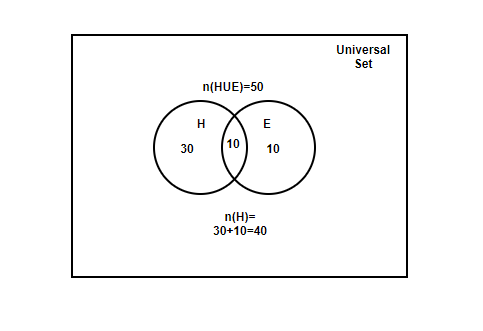Question 3: In a Class, Students like to play these games- Football, Cricket, Volleyball. 5 students Play all 3 games, 20 play Football, 30 play Volleyball, 40 play Cricket. 10 play both cricket and volleyball, 12 play both football and cricket, 9 play both football and volleyball. How many students are present in the class?

Solution:

n(F∪ C∪ V)= n(F)+ n(C)+ n(V) – n(F∩C) – n(F∩V) – n(C∩V)+ n(F∩ C∩ V)

n(F∪ C∪ V)= 20+ 30+ 40- 10-12-9+5

n(F∪ C∪ V)= 64

There are 64 Students in the class.

Question 4: Represent the above information with the help of a Venn diagram showing the amount of data present in each set.

The above information should look something like this on Venn diagram,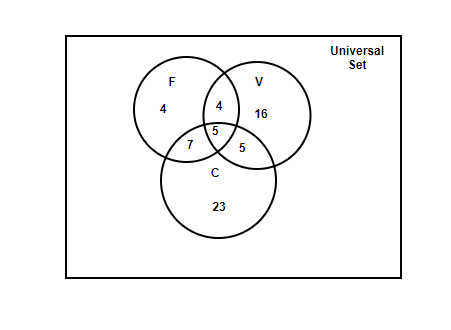Question 5: Below given Venn diagram has all the sufficient information required to show the data of all the sets possible. Observe the diagram carefully then answer the following.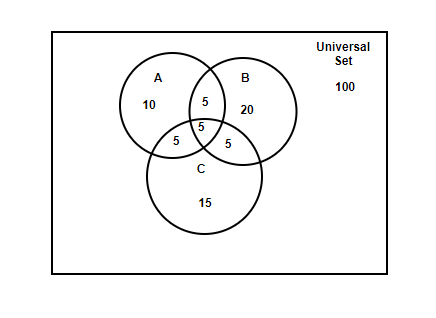1. What is the value of n(A∩ B∩ C)?

2. What is the value of n(C)?

3. What is the value of n(B ∩ A)?

4. What is the value of n(A∪ B∪ C)?

5. What is the value of n(B’)?

Observing the Venn diagram, the above questions can be easily answered,

1. n(A ∩ B∩ C)= 5

2. n(C)= 15+ 5+5+5= 30

3. n( B∩A)= 5+5= 10

4. n(A∪ B∪ C)= 15+ 20+ 10+ 5+ 5+ 5+ 5= 65

5. n(B’)= U- n(B)= 100- (20+ 5+ 5+ 5)= 100- 35= 65

Attention reader! Don’t stop learning now. Join the First-Step-to-DSA Course for Class 9 to 12 students , specifically designed to introduce data structures and algorithms to the class 9 to 12 students

My Personal Notes arrow_drop_up